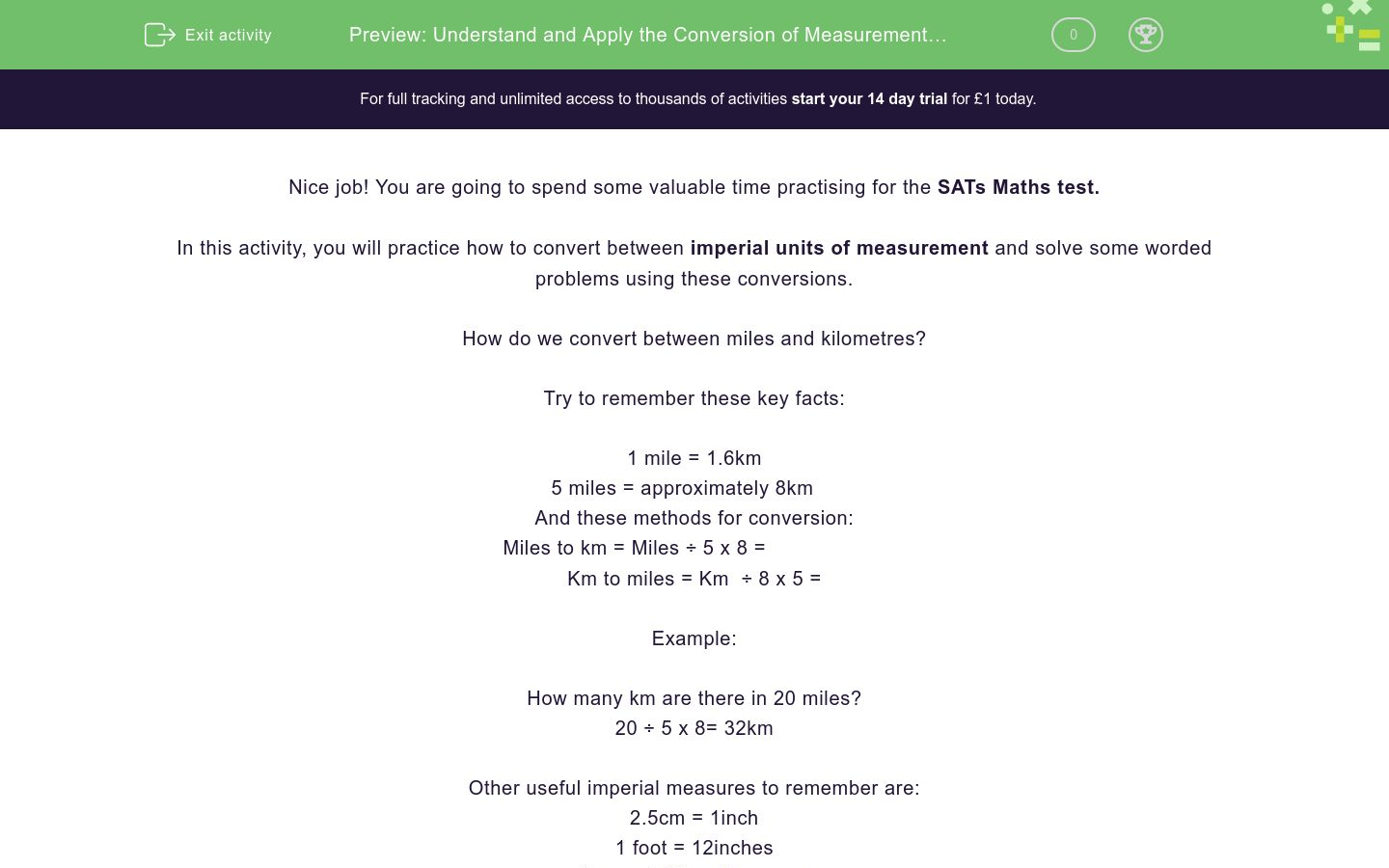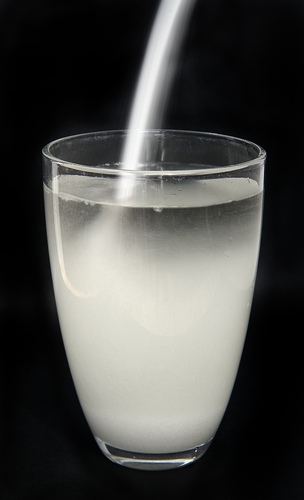# Understand and Apply the Conversion of Measurement Units 2

In this worksheet, students will be asked to convert imperial units of measurement. The student will also have to use reasoning skills to solve some worded problems involving measurement.Key stage:  KS 2

Curriculum topic:   Exam-style Questions: SATs Maths

Curriculum subtopic:   Exam-Style Questions: Decimal Conversion

Difficulty level:### QUESTION 1 of 10

Nice job! You are going to spend some valuable time practising for the SATs Maths test.

In this activity, you will practice how to convert between imperial units of measurement and solve some worded problems using these conversions.

How do we convert between miles and kilometres?

Try to remember these key facts:

1 mile = 1.6km

5 miles = approximately 8km

And these methods for conversion:

Miles to km = Miles ÷ 5 x 8 =​

Km to miles = Km  ÷ 8 x 5 =

Example:

How many km are there in 20 miles?

20 ÷ ​5 x 8= 32km

Other useful imperial measures to remember are:

2.5cm = 1inch

1 foot = 12inches

1 pound (lb) = 16 ounces

1 stone = 14 pounds(lbs)

1 gallon = 8 pints

Over to you now to have a go at some practice questions, use all of the information given to help you!

5 miles = approximately 8 km

How many kilometres are there in 40 miles?

60 km

62 km

61 km

64 km

5 miles = approximately 8 km

How many miles are there in 200 km?

105 miles

125 miles

115 miles

120 miles

Can you match the equivalent units of measure?

Remember...

5 miles = approximately 8 km

## Column B

120 km =
56 km
35 miles =
30 miles
60 miles =
75 miles
48 km =
96 m

Read and solve this worded problem...

Today I drove 20 miles to Birmingham and then a further 40 miles to Leicester.

How far did I travel in km?## Column B

120 km =
56 km
35 miles =
30 miles
60 miles =
75 miles
48 km =
96 m

If 2.5 cm = 1 inch, how many inches is 25 cm worth?## Column B

120 km =
56 km
35 miles =
30 miles
60 miles =
75 miles
48 km =
96 m

Now, let's try converting feet and inches.

1 foot = approximately 12 inches.

How many inches are there in 8 ft?

84 in

102 in

64 in

96 in

1 pound (lb) = approximately 16 ounces

## Column B

1000 lbs =
20 lbs
2000 lbs =
96 ounces
6 lbs =
32,000 ounces
320 ounces =
16,000 ounces

14 pounds (lb) = approximately 1 stone

## Column B

11 stones =
7 lbs
112 lbs =
8 stones
42 lbs=
154 lbs
0.5 stones =
3 stones

1 gallon = 8 pints

Over 4 days, Ted drinks 4 pints of water a day.

How much does he drink in total? Give your answer in gallons.## Column B

11 stones =
7 lbs
112 lbs =
8 stones
42 lbs=
154 lbs
0.5 stones =
3 stones

1 stone = approximately 14 lbs

Two friends decide to weigh themselves.

Lindsay weighs 42 lbs

Tilly weighs 56 lbs

What is the difference between their weight?

## Column B

11 stones =
7 lbs
112 lbs =
8 stones
42 lbs=
154 lbs
0.5 stones =
3 stones
• Question 1

5 miles = approximately 8 km

How many kilometres are there in 40 miles?

64 km
EDDIE SAYS
Great start if you chose 64 miles. To solve this one we had to remember that to convert to km, we have to divide by 5 and multiply by 8. 40÷5 = 8 x 8 = 64 km
• Question 2

5 miles = approximately 8 km

How many miles are there in 200 km?

125 miles
EDDIE SAYS
Nice job if you chose 125 miles. To crack this second question, we had to remember to ÷8 and x 5. 200 ÷ 8 = 25 x 5 = 125 miles This is a good start- let's keep up the effort!
• Question 3

Can you match the equivalent units of measure?

Remember...

5 miles = approximately 8 km

## Column B

120 km =
75 miles
35 miles =
56 km
60 miles =
96 m
48 km =
30 miles
EDDIE SAYS
Phew, that was a toughie- how did you do? To calculate the matching pairs of imperial units, you needed to remember the two methods for conversion: Miles to km= miles ÷ 5 x 8 Km to miles = km ÷8 x 5
• Question 4

Read and solve this worded problem...

Today I drove 20 miles to Birmingham and then a further 40 miles to Leicester.

How far did I travel in km?EDDIE SAYS
Keep going until you get it right! To solve this question, we had to follow these steps: Step 1: add the total miles together: 20+40 =60 miles Step 2: 60 miles ÷ 5 = 12 x 8 = 96 km You are making great progress- keep it up!
• Question 5

If 2.5 cm = 1 inch, how many inches is 25 cm worth?EDDIE SAYS
Did you see that if 2.5 cm = 1 inch, 25 cm will be worth 10 times the number of inches, so 10 inches? 1 x 10 = 10 Keep up your hard work, you are halfway through now!
• Question 6

Now, let's try converting feet and inches.

1 foot = approximately 12 inches.

How many inches are there in 8 ft?

96 in
EDDIE SAYS
Nice job if you chose 96 in. We were told that 1ft = 12 inches To find how many inches are in 8ft, we multiply 12 by 8. 12 x 8 = 96 in Remember that feet and inches are a type of imperial measure unit, an old-fashioned type of measurement. The more modern metric unit for this type of measurement would be cm and m.
• Question 7

1 pound (lb) = approximately 16 ounces

## Column B

1000 lbs =
16,000 ounces
2000 lbs =
32,000 ounces
6 lbs =
96 ounces
320 ounces =
20 lbs
EDDIE SAYS
Don't panic if you found this difficult, there was a lot to think about here! When converting from lbs to ounces, multiply by 16 When converting from ounces to lbs, divide by 16 Use whichever method you feel comfortable with to work out the answer, for example, bus stop method for division or grid method for multiplication, but remember, you will not be allowed a calculator in the test!
• Question 8

14 pounds (lb) = approximately 1 stone

## Column B

11 stones =
154 lbs
112 lbs =
8 stones
42 lbs=
3 stones
0.5 stones =
7 lbs
EDDIE SAYS
Don't be afraid to give it a go! The more you try, the more you'll know! When converting from lbs to stones, divide by 14 When converting from stones to lbs, multiply by 14 Wow, you only have 2 questions left, keep going!
• Question 9

1 gallon = 8 pints

Over 4 days, Ted drinks 4 pints of water a day.

How much does he drink in total? Give your answer in gallons.EDDIE SAYS
Did you manage to work out that Ted drank 2 gallons of water over 4 days? To solve this problem: Step 1: Work out the total amount of water drank: 4 x 4 = 16 pints Step 2: Divide 16 pints by 8 to find out the number of gallons: 16 pints ÷ 8 = 2 gallons You have made a super effort; one more question to go!
• Question 10

1 stone = approximately 14 lbs

Two friends decide to weigh themselves.

Lindsay weighs 42 lbs

Tilly weighs 56 lbs

What is the difference between their weight?

EDDIE SAYS
Did you find that the difference between the girl's weight is 1 stone? To find this answer, we had to first convert each girl's weight into stones. Lindsay: 42 ÷ 14 = 3 stones Tilly: 56 ÷ 14 = 4 stones We can then clearly see that there is a 1 stone difference between their weights. Well done, that's another activity completed!